zhangyiqun

Thoughts, stories and ideas.

# 27 Feb 2020 Tensor

## 基本操作

``````tensor(im3)[4:10,4:10]
``````

tensor生成

``````# 1D tensor
x = torch.empty(3)
print(x)

# 2D tensor
x = torch.empty(3,3)
print(x)

# 3d tensor
x = torch.empty(3,3,3)
print(x)
``````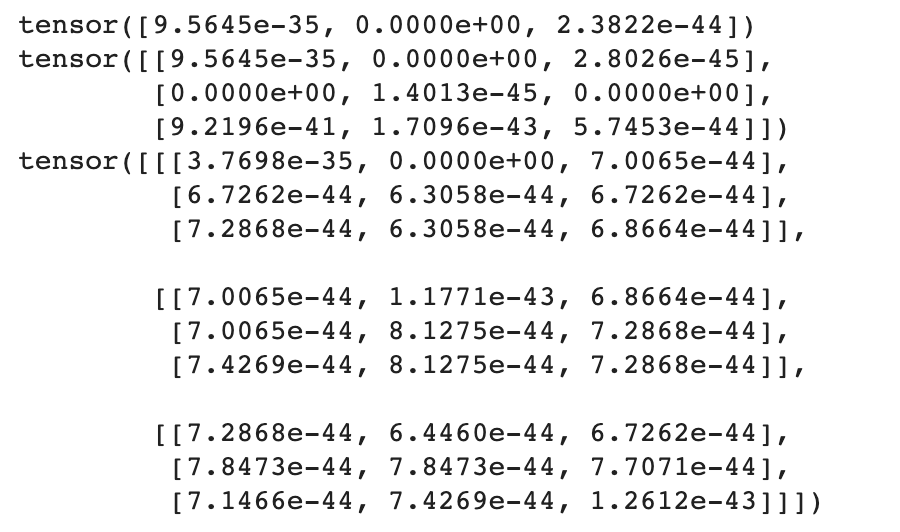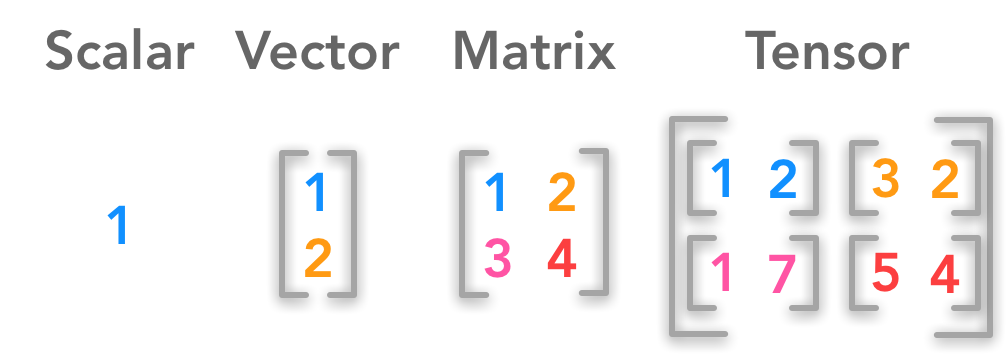But in the world of deep learning, it’s actually not scary at all. “Tensor” means array, but specifically it’s an array of a regular shape. So it’s not an array where row 1 has two things, row 3 has three things, and row 4 has one thing, what you call a “jagged array”. That’s not a tensor. A tensor is any array which has a rectangular or cube or whatever ﹣ a shape where every row is the same length and every column is the same length.

https://hadrienj.github.io/posts/Deep-Learning-Book-Series-2.1-Scalars-Vectors-Matrices-and-Tensors

## Rank

We don’t say dimensions, though, with tensors. We use one of two words, we either say rank or axis. Rank specifically means how many axes are there, how many dimensions are there.

## 数学名词很多

But in math, people come up with very different words for slightly different concepts. Why is a one dimensional array a vector and a two dimensional array is a matrix, and a three dimensional array doesn’t have a name. It doesn’t make any sense. With computers, we try to have some simple consistent naming conventions. They are all called tensors﹣rank 1 tensor, rank 2 tensor, rank 3 tensor. You can certainly have a rank 4 tensor. If you’ve got 64 images, then that would be a rank 4 tensor of 64 by 480 by 640 by 3. So tensors are very simple. They just mean arrays.

## 2D Color Image = 3D Tensor

``````seven_tensors = [tensor(Image.open(o)) for o in sevens]
three_tensors = [tensor(Image.open(o)) for o in threes]

stacked_sevens = torch.stack(seven_tensors).float()/255
stacked_threes = torch.stack(three_tensors).float()/255
``````

2D Tensor如何存储 3 x 3 的表格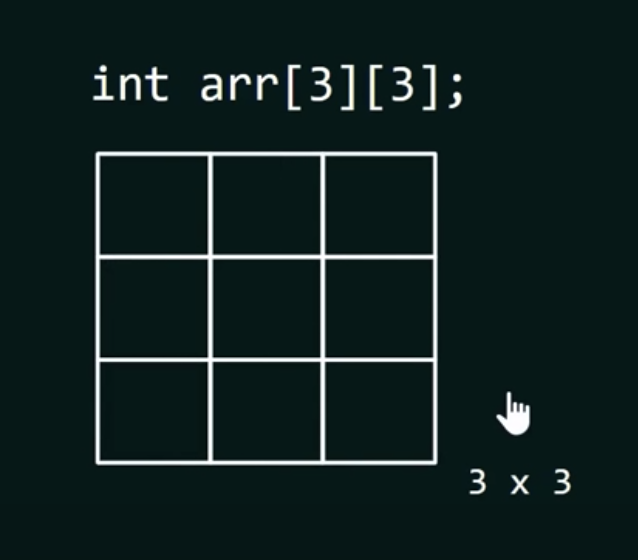3D Tensor如何存 3 x 3 的表格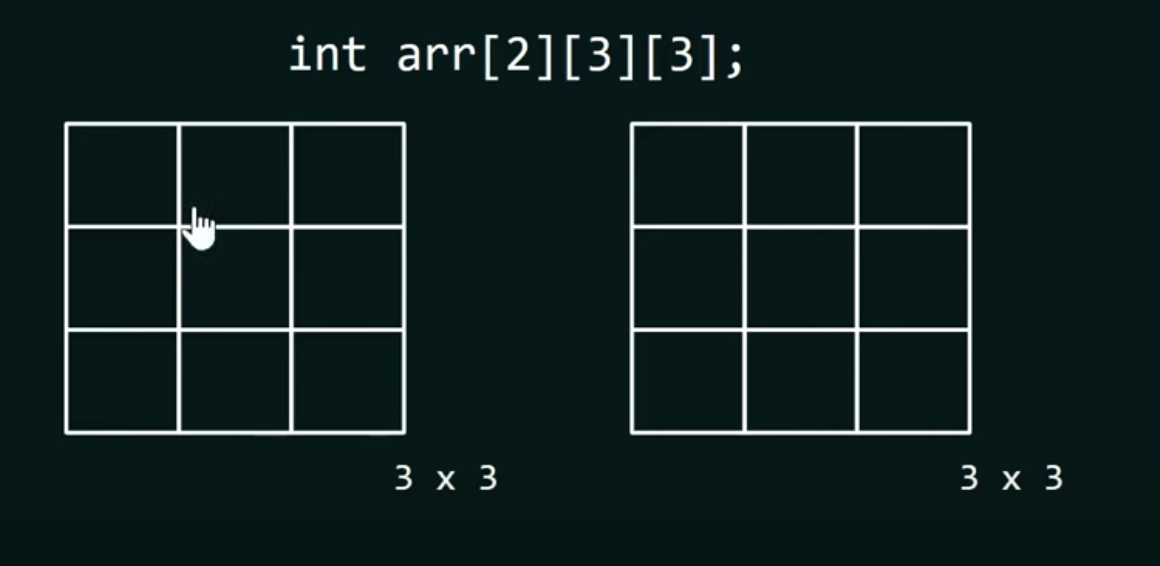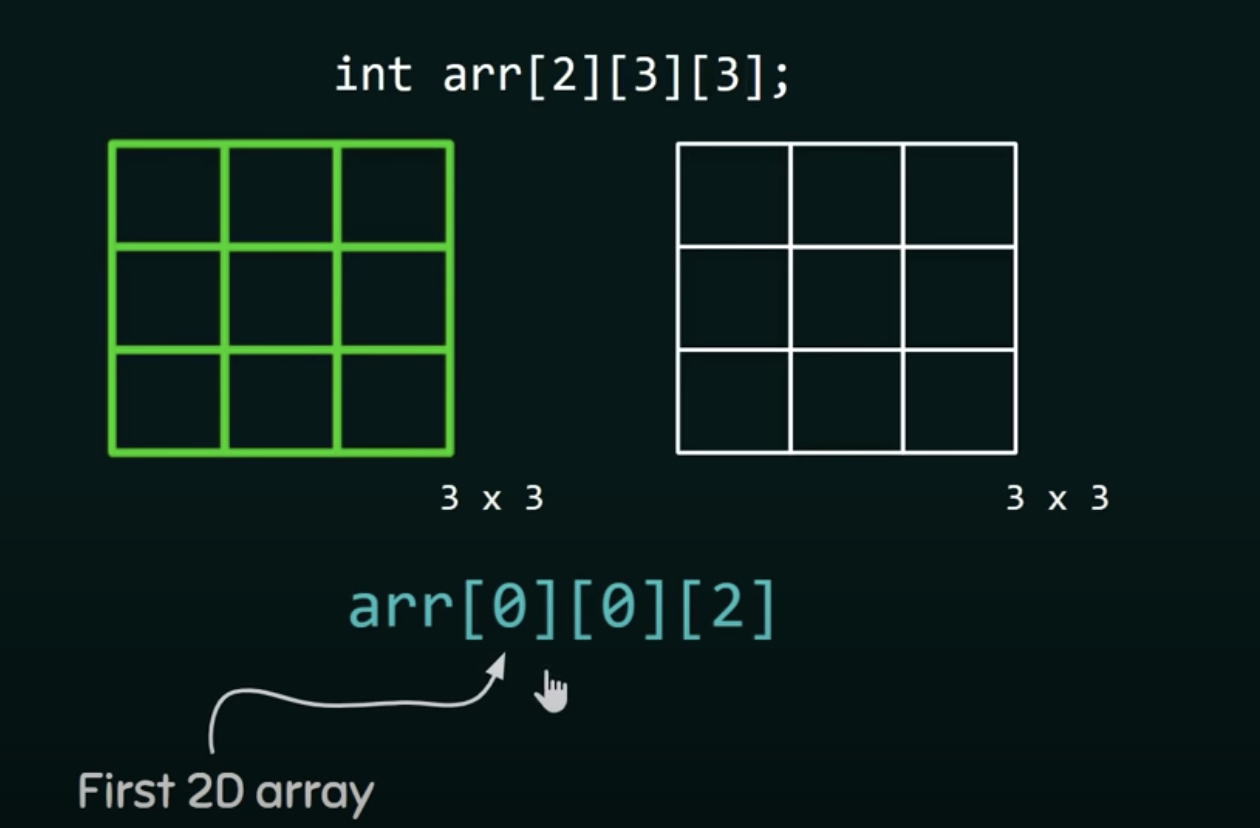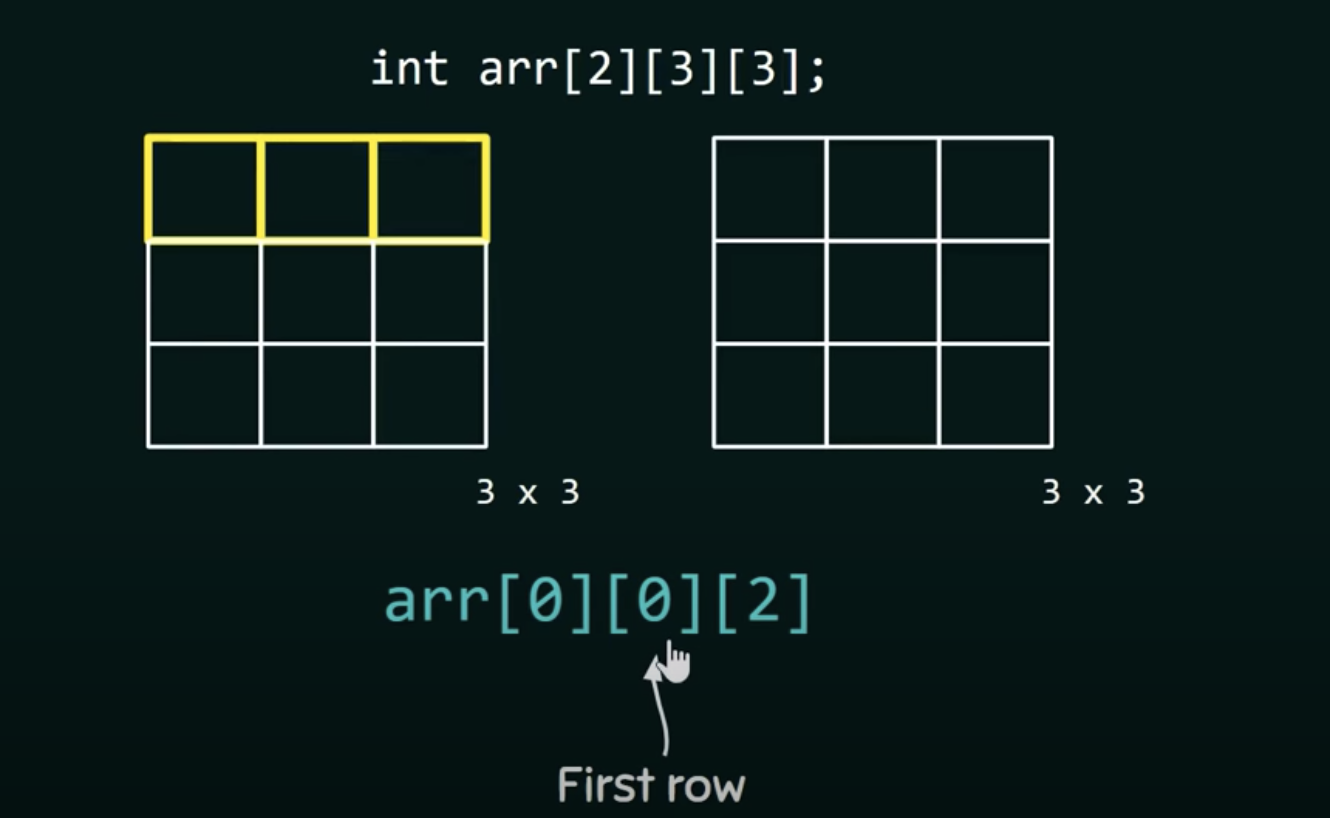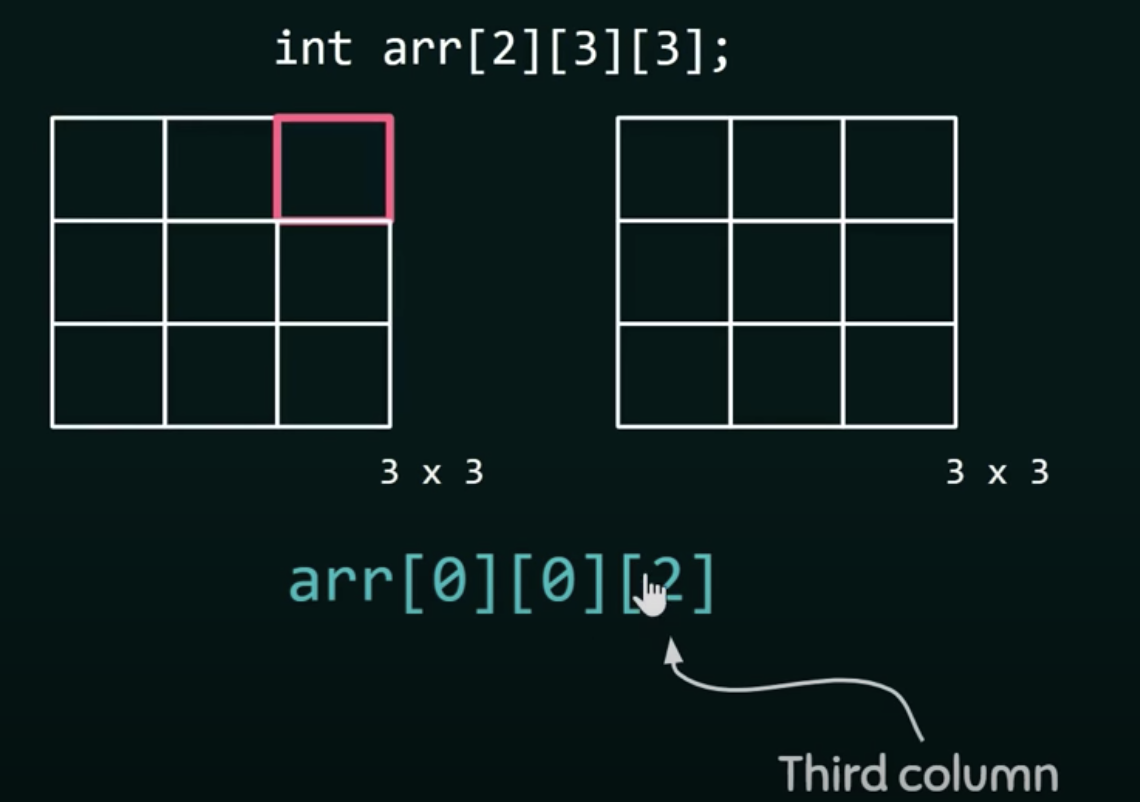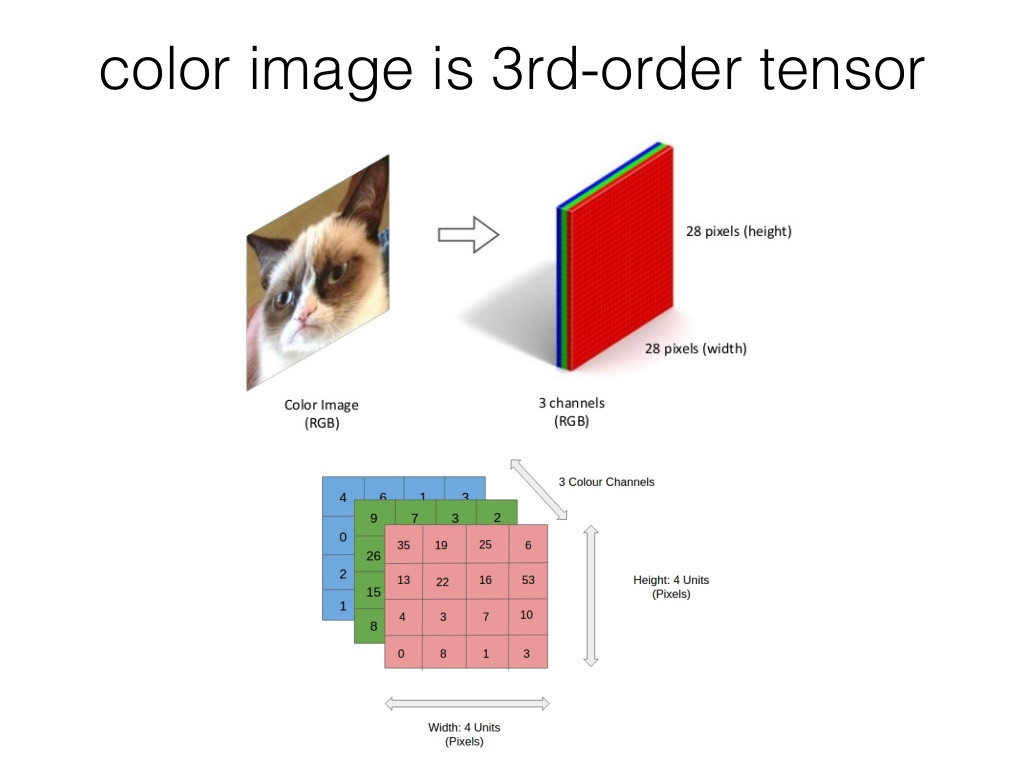You can certainly have a rank 4 tensor. If you’ve got 64 images, then that would be a rank 4 tensor of 64 by 480 by 640 by 3. So tensors are very simple. They just mean arrays.

https://lisaong.github.io/mldds-courseware/01_GettingStarted/numpy-tensor-slicing.slides.html